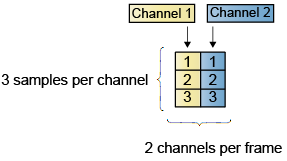Documentation

This is machine translation

Mouseover text to see original. Click the button below to return to the English version of the page.

Create Multichannel Signals for Frame-Based Processing

In frame-based processing, blocks process data one frame at a time. Each frame of data contains sequential samples from an independent channel. Each channel is represented by a column of the input signal. For example, from a frame-based processing perspective, the following 3-by-2 matrix has two channels, each of which contains three samples.When you configure a block to perform frame-based processing, the block interprets an M-by-1 vector as a single-channel signal containing M samples per frame. Similarly, the block interprets an M-by-N matrix as a multichannel signal with N independent channels and M samples per channel. For example, in frame-based processing, blocks interpret the following sequence of 3-by-2 matrices as a two-channel signal with a frame size of 3.Using frame-based processing is advantageous for many signal processing applications because you can process multiple samples at once. By buffering your data into frames and processing multisample frames of data, you can often improve the computational time of your signal processing algorithms. To perform frame-based processing, you must have a DSP System Toolbox™ license.

For more information about the recent changes to frame-based processing, see the Frame-based processing changes section of the DSP System Toolbox Release Notes.

Multichannel Signals for Frame-Based Processing

When you want to perform the same operations on several independent signals, you can group those signals together as a multichannel signal. For example, if you need to filter each of four independent signals using the same direct-form II transposed filter, you can combine the signals into a multichannel signal, and connect the signal to a single Biquad Filter block. The block decides to treat each column of the input as a channel when you set the block's Input processing parameter to ```Columns as channels (frame based)```. The block then applies the filter to each channel independently.

A signal with N channels and frame size M is represented by a matrix of size M-by-N. Multiple individual signals with the same frame rate and frame size can be combined into a single multichannel signal using the Simulink® Matrix Concatenate block. Individual signals can be added to an existing multichannel signal in the same way.Create Multichannel Signals Using Concatenate Block

You can combine independent signals into a larger multichannel signal by using the Simulink Concatenate block. All signals must have the same frame rate and frame size. In this example, a single-channel signal is combined with a two-channel signal to produce a three-channel signal:

1. Open the Matrix Concatenate Example 3 model by typing

`ex_combiningfbsigs`

at the MATLAB® command line.2. Double-click the Signal From Workspace block. Set the block parameters as follows:

• Signal = `[1:10;-1:-1:-10]'`

• Sample time = `1`

• Samples per frame = `4`

Based on these parameters, the Signal From Workspace block outputs a signal with a frame size of four.

3. Save these parameters and close the dialog box by clicking .

4. Double-click the Signal From Workspace1 block. Set the block parameters as follows, and then click :

• Signal = `5*ones(10,1)`

• Sample time = `1`

• Samples per frame = `4`

The Signal From Workspace1 block has the same sample time and frame size as the Signal From Workspace block. To combine single-channel signals into a multichannel signal, the signals must have the same frame rate and the same frame size.

5. Double-click the Matrix Concatenate block. Set the block parameters as follows, and then click :

• Number of inputs = `2`

• Mode = ```Multidimensional array```

• Concatenate dimension = `2`

6. Run the model.

The 4-by-3 matrix output from the Matrix Concatenate block contains all three input channels, and preserves their common frame rate and frame size.

Watch now